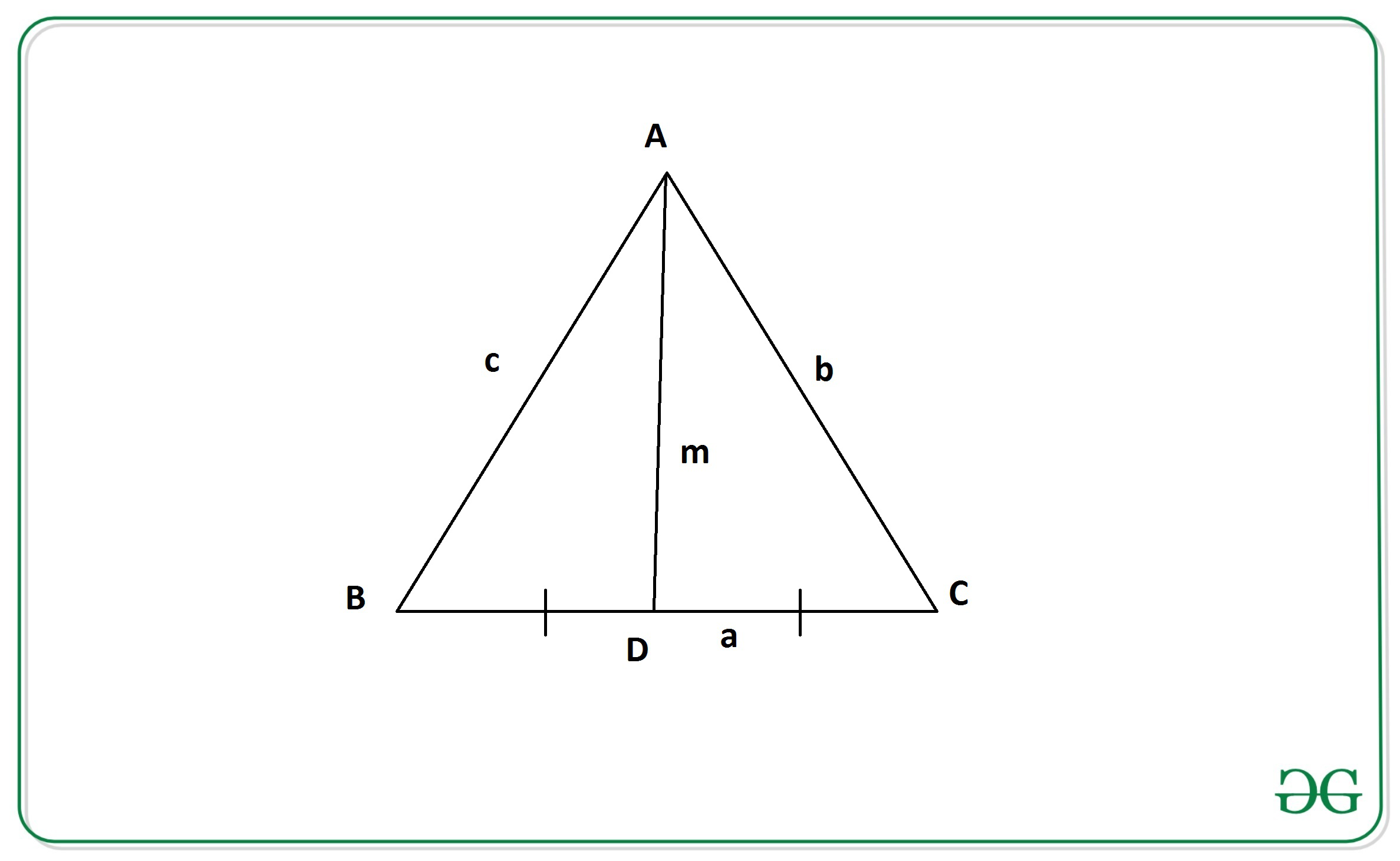Open in App
Not now

# Find the length of the median of a Triangle if length of sides are given

• Difficulty Level : Easy
• Last Updated : 27 Aug, 2022

Given the length of all three sides of a triangle as a, b and c. The task is to calculate the length of the median of the triangle.

A median of a triangle is a line segment joining a vertex to the midpoint of the opposite side, thus bisecting that side.

Examples:

Input: a = 8, b = 10, c = 13
Output: 10.89
Input: a = 4, b = 3, c = 5
Output: 3.61

Approach: The idea is to use Apollonius’s theorem to solve this problem.

Apollonius’s Theorem states that “the sum of the squares of any two sides of a triangle equals twice the square on half the third side and twice the square on the median bisecting the third side”.From the above figure, According to Apollonius’s Theorem we have:where a, b, and c are the length of sides of the triangle
and m is the length of median of the triangle on side 2*a

Therefore, the length of the median of a triangle from the above equation is given by:Below is the implementation of the above approach:

## C++

 // C++ program to find the length of the  // median using sides of the triangle #include using namespace std;   // Function to return the length of // the median using sides of triangle float median(int a, int b, int c) {     float n = sqrt(2 * b * b +                     2 * c * c - a * a) / 2;     return n; }   // Driver code int main() {     int a, b, c;     a = 4;     b = 3;     c = 5;       // Function call     float ans = median(a, b, c);        // Print final answer with 2      // digits after decimal     cout << fixed << setprecision(2) << ans;     return 0; }   // This code is contributed by himanshu77

## Java

 // Java program to find the length of the  // median using sides of the triangle  import java.util.*;    class GFG{       // Function to return the length of  // the median using sides of triangle  public static float median(int a, int b, int c)  {      float n = (float)(Math.sqrt(2 * b * b +                                  2 * c * c -                                  a * a) / 2);      return n;  }    // Driver code public static void main(String[] args) {     int a, b, c;      a = 4;      b = 3;      c = 5;        // Function call      float ans = median(a, b, c);        // Print final answer with 2      // digits after decimal      System.out.println(String.format("%.2f", ans));  } }   // This code is contributed by divyeshrabadiya07

## Python3

 # Python3 implementation to Find the  # length of the median using sides # of the triangle   import math   # Function to return the length of  # the median using sides of triangle.  def median(a, b, c):         n = (1 / 2)*math.sqrt(2*(b**2)     + 2*(c**2)  - a**2)         return n    # Driver Code  a = 4 b = 3 c = 5   # Function Call ans = median(a, b, c)   # Print the final answer print(round(ans, 2))

## C#

 // C# program to find the length of the  // median using sides of the triangle  using System;   class GFG{       // Function to return the length of  // the median using sides of triangle  public static float median(int a, int b, int c)  {      float n = (float)(Math.Sqrt(2 * b * b +                                  2 * c * c -                                  a * a) / 2);      return n;  }    // Driver code public static void Main(String[] args) {     int a, b, c;      a = 4;      b = 3;      c = 5;        // Function call      float ans = median(a, b, c);        // Print readonly answer with 2      // digits after decimal      Console.WriteLine(String.Format("{0:F2}", ans));  } }   // This code is contributed by gauravrajput1

## Javascript

 

Output:

3.61

Time Complexity: O(log(n)) because using inbuilt sqrt function
Space Complexity: O(1)

My Personal Notes arrow_drop_up
Related Articles